# Numbers

Natural numbers The counting numbers 1,2,3,4 . . . are called natural numbers.

Whole number All natural numbers including zero are called whole numbers.
Properties of whole number:-

Addition– closure property- the sum of whole numbers is always a whole number.
Commutative property- a+b = b+a
Associative property- (a+b)+c = a+(b+c)
Identity element- if zero is added to any whole number the sum is the number itself.
0+a = a+0 = a
subtraction – closure property- the difference of two whole numbers is not necessary a whole number a-b ≠ b-a not necessary a whole number.
Commutative property a-b ≠ b-a is not defined.
12-4 ≠ 4-12 is not defined.
Property of zero- 0-a ≠ 0-b is not a defined.
Associative property- (a-b)-c ≠ a-(b-c)

Division– Dividend- the number which is to be divided is called dividend.
Divisor– the number by which dividend is divided is called the divisor.
Quotient– the number of times the divisor is contained in the dividend is called the quotient.
Reminder– the left over number after division is called the reminder.
Thus, the relationship between these terms.Integer All whole numbers along with negatives of natural numbers are called integers.

Fractions The numbers in the form of a/b where a and b are whole numbers and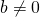Rational numbers The numbers in the form of a/b a where a and b are integers.
Fractions are also rational numbers a is called numerator and b is called denominator
equivalent rational number.

Relational numbers between two relational numbers

If given two relational numbers are a and b then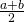is relational number between a and b.
ExampleandMore that one relational numbers between two rational numbers
Write and rational numbers betweenandConvert two given rational number into equivalent form with common denominatorTo obtain 4 Relational numbers between them multiply numerator and denominators with (4+1=5) to make equivalent relational numbers(Multiply n+1 times where n is required number of relational number between two relational number)andthen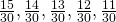and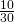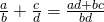where the denominator of Relational numbers is greater.

Relational numbers multiplication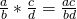Relational numbers divisionFactor– A factor of a number is an exact divisor of the number. Itself in other word the number that are multiplied to get a product are called the factor . for e.g.- 1,2,3,4,6&12 are the factors of 12.
Multiple- a multiple of a number is a number obtained by multiplying it by a natural number. If we multiply 4 by 1,2,3 we get 4,8, 12 which we all multiple of 4.

Even number– A natural number which is exactly divided by 2 is called an even number.
Odd number– A natural number which is not exactly divisible by 2 is called an odd number.
Prime number– A natural number which is greater than 1 & whose only factors are 1 and the number itself is a prime number.
Composite number– The number having more than two factors are called composite numbers.
Co-prime numbers– The two numbers which has no common factor other than one are called co-prime number (2,3) (3,4)